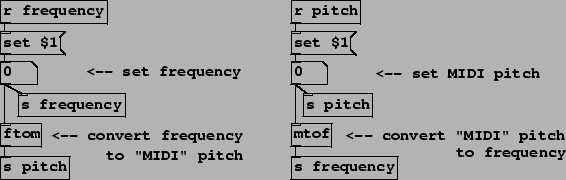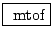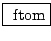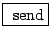Conversion between frequency and pitch

Example A06.frequency.pd  (Figure 1.13) shows Pd's object for converting pitch to frequency units (mtof, meaning MIDI to frequency") and its inverse ftom. We also introduce two other object classes, send and receive.,: convert MIDI pitch to frequency units according to the Pitch/Frequency Conversion Formulas (Page). Inputs and outputs are messages (tilde" equivalents of the two also exist, although like dbtorms~ they're expensive in CPU time). The ftom object's output is -1500 if the input is zero or negative; and likewise, if you give mtof -1500 or lower it outputs zero.,: Receive messages non-locally. The receive object, which may be abbreviated as r", waits for non-local messages to be sent by a send object (described below) or by a message box using redirection (the ;" feature discussed in the earlier example, A01.sinewave.pd). The argument (such as frequency" and pitch" in this example) is the name to which messages are sent. Multiple receive objects may share the same name, in which case any message sent to that name will go to all of them.,: The send object, which may be abbreviated as s", directs messages to receive objects.

Two new properties of number boxes are used here. Earlier we've used them as controls or as displays; here, the two number boxes each function as both. If a number box gets a number in its inlet, it not only displays the number but also repeats the number to its output. However, a number box may also be sent a set" message, such as set 55" for example. This would set the value of the number box to 55 (and display it) but not cause the output that would result from the simple 55" message. In this case, numbers coming from the two receive objects are formatted (using message boxes) to read set 55" instead of just 55", and so on. (The special word \$1" is replaced by the incoming number.) This is done because otherwise we would have an infinite loop: frequency would change pitch which would change frequency and so on forever, or at least until something broke.